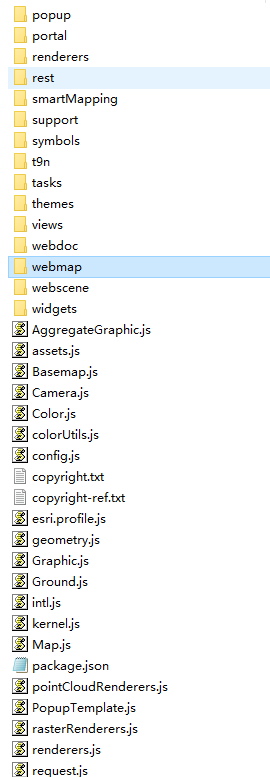+关注继续查看

# 用R画地图数据library(maptools)
plot(mydat)install.packages(mapdata)
install.packages("mapdata")
library(maps)
library(mapdata)
map("china")map(database = "world", regions = ".", exact = FALSE, boundary = TRUE,
interior = TRUE, projection = "", parameters = NULL, orientation = NULL,
fill = FALSE, col = 1, plot = TRUE, add = FALSE, namesonly = FALSE,
xlim = NULL, ylim = NULL, wrap = FALSE, resolution = if (plot) 1 else 0,
type = "l", bg = par("bg"), mar = c(4.1, 4.1, par("mar"), 0.1),
myborder = 0.01, ...)

# 给地图上色

plot命令中的fg参数在本例中应该是一个长度为925的向量，其第i个分量的取值就代表了地图中第i个多边形的颜色。一个简单的尝试是运行下面这个命令看看效果：

plot(x,col=gray(924:0/924));col=c(othercol,provcol)[colIndex+1];
return(col);
}

provname=c("北京市","天津市","上海市","重庆市");
provcol=c("red","green","yellow","purple");
plot(x,col=getColor(x,provname,provcol,"white"));# 生成人口数据分布图

provname=c("北京市","天津市","河北省","山西省","内蒙古自治区",
"辽宁省","吉林省","黑龙江省","上海市","江苏省",
"浙江省","安徽省","福建省","江西省","山东省",
"河南省","湖北省","湖南省","广东省",
"广西壮族自治区","海南省","重庆市","四川省","贵州省",
"云南省","西藏自治区","陕西省","甘肃省","青海省",
"宁夏回族自治区","新疆维吾尔自治区","台湾省",
"香港特别行政区");
pop=c(1633,1115,6943,3393,2405,4298,2730,3824,1858,7625,
5060,6118,3581,4368,9367,9360,5699,6355,9449,
4768,845,2816,8127,3762,4514,284,3748,2617,
552,610,2095,2296,693);
provcol=rgb(red=1-pop/max(pop)/2,green=1-pop/max(pop)/2,blue=0);
plot(x,col=getColor(x,provname,provcol,"white"),xlab="",ylab="");# 画部分省地图

getID=function(mapdata,provname)
{
index=mapdata$att.data$NAME %in% provname;
ids=rownames(mapdata$att.data[index,]); return(as.numeric(ids)); } midchina=c("河南省","山西省","湖北省","安徽省","湖南省","江西省"); plot(x, col = getColor(x, midchina, rep("green", 6), "white"), border = "white", xlab = "", ylab = "") 生成的图：# 用R画中国地图并标注城市位置 画出的图上仍然可以用points()函数和text()函数加上点和文字，而maptools包中还提供了一个pointLabel()函数，用来解决文本标签的重叠问题。 par(mar=rep(0,4)) dat = read.csv(text = "城市,jd,wd 北 京,116.4666667,39.9 上 海,121.4833333,31.23333333 天 津,117.1833333,39.15 重 庆,106.5333333,29.53333333 哈尔滨,126.6833333,45.75 长 春,125.3166667,43.86666667 沈 阳,123.4,41.83333333 呼和浩特,111.8,40.81666667 石家庄,114.4666667,38.03333333 太 原,112.5666667,37.86666667 济 南,117,36.63333333 郑 州,113.7,34.8 西 安,108.9,34.26666667 兰 州,103.8166667,36.05 银 川,106.2666667,38.33333333 西 宁,101.75,36.63333333 乌鲁木齐,87.6,43.8 合 肥,117.3,31.85 南 京,118.8333333,32.03333333 杭 州,120.15,30.23333333 长 沙,113,28.18333333 南 昌,115.8666667,28.68333333 武 汉,114.35,30.61666667 成 都,104.0833333,30.65 贵 阳,106.7,26.58333333 福 州,119.3,26.08333333 台 北,121.5166667,25.05 广 州,113.25,23.13333333 海 口,110.3333333,20.03333333 南 宁,108.3333333,22.8 昆 明,102.6833333,25 拉 萨,91.16666667,29.66666667 香 港,114.1666667,22.3 澳门,113.5,22.2") library(maps) library(mapdata) map("china", col = "darkgray", ylim = c(18, 54), panel.first = grid()) points(dat$jd, dat$wd, pch = 19, col = rgb(0, 0, 0, 0.5)) text(dat$jd, dat$wd, dat[, 1], cex = 0.9, col = rgb(0, 0, 0, 0.7), pos = c(2, 4, 4, 4, 3, 4, 2, 3, 4, 2, 4, 2, 2, 4, 3, 2, 1, 3, 1, 1, 2, 3, 2, 2, 1, 2, 4, 3, 1, 2, 2, 4, 4, 2)) axis(1, lwd = 0); axis(2, lwd = 0); axis(3, lwd = 0); axis(4, lwd = 0) 生成的图：# ggplot2绘制地图 以中国地图为例，下载最新的ArcGIS矢量地图数据，这种地图数据包含了很多信息，这是画地图的基础数据。下载地址： http://download.csdn.net/detail/lgstarzkhl/9427677 用以下代码进行地图绘制： library(maptools) library(ggplot2) library(plyr) #读取地图文件 china_map<-readShapePoly("C:/Users/feng/Desktop/chinaprovinceborderdata_tar_gz/china-province-border-data.tar/Lambert/省级行政区.shp") #提取用于绘图的地图数据 x<-china_map@data xs<-data.frame(x,id.1=seq(0:33)-1) #将地图数据转换为数据框 china_map1<-fortify(china_map) #添加一个id.1字段，用于和上面的xs（各省市数据）糅合，合并 china_map1$id.1<-china_map1\$id
#去掉china_map1中的id字段，避免在糅合数据的时候，出现两个相同字段id和id.1，保证只用id.1来糅合
china_map2<-china_map1[,-7]
#糅合地图数据
china_mapdata<-join(china_map2, xs, type = "full")
#绘制地图
ggplot(china_mapdata, aes(x = long, y = lat, group = group,fill=NAME))+
geom_polygon( )+
geom_path(colour = "grey40")+
scale_fill_manual(values=colours(),guide=FALSE)# 总结|
2月前
|

236 0
|
2月前
|

128 0
|
2月前
|

41 0
|
JSON 数据可视化 JavaScript
echars地图实现下钻

401 0
|
JavaScript 前端开发 程序员

153 0|

GIS地图瓦片之缩放级别原理

1494 0
|
JavaScript 定位技术 容器
【D3.js 学习总结】26、D3地理地图
# d3.geo ![](https://img.alicdn.com/tps/TB1M2eWLFXXXXcXXFXXXXXXXXXX-920-688.png) 在学习D3如何制作地图前，我们需要了解下地图的数据格式geoJSON，GeoJSON是一种对各种地理数据结构进行编码的格式，基于Javascript对象表示法的地理空间信息数据交换格式。 本节我们将制作一幅中国地图，使用
2460 0